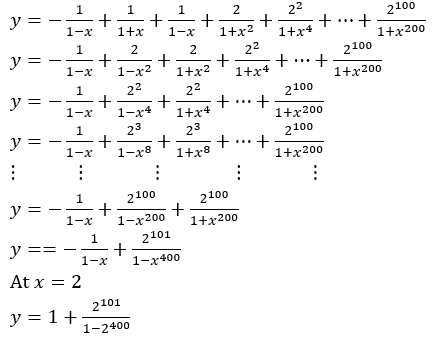Win up to 100% scholarship on Aakash BYJU'S JEE/NEET courses with ABNAT Win up to 100% scholarship on Aakash BYJU'S JEE/NEET courses with ABNAT

# JEE Main 2021 August 26 Shift 1 Maths Question Paper with Solutions

JEE Main Question Paper solutions for August 26 Shift 1 Maths are available here. Candidates can find the JEE Main 2021 August 26 Shift 1 Maths Question Paper with Solutions to evaluate their performance. Students can directly download the PDF version of the question paper to check solutions offline. This set of solved questions given in JEE Main 2021 session 4 Maths question paper for August 26 will help you to formulate the correct answers. The JEE Main 2021 Shift 1 Maths question paper for August 26 given here will aid students to eventually be well prepared to face the upcoming exam.

JEE Main 2021 August 26th Shift 1 Maths Question Paper

Question 1. f(x) =

$$\begin{array}{l}cos((2 tan^{-1}((sin((cot^{-1}(\sqrt{((1-x)/x) )} ) )\end{array}$$
then;

a. (1-x)2 f'(x) – 2(f(x)) 2 = 0
b. (1-x) 2 f'(x) + 2(f(x)) 2= 0
c. (1+x) 2 f'(x) – 2(f(x)) 2= 0
d. (1+x) 2 f'(x)+2(f(x)) 2= 0

f(x) =

$$\begin{array}{l}cos((2 tan^{-1}((sin((cot^{-1}(\sqrt{((1-x)/x) )} ) )\end{array}$$
then

f(x) =

$$\begin{array}{l}cos((2 tan^{-1}((sin((sin^{-1}(\sqrt{x} ) ) ) \end{array}$$

= cos((2 tan-1(√x)

= cos((cos-1 (((1 – x)/(1 + x)) ) = (1 – x)/(1 + x)

f'(x) = -2/(1+x)2

2(f(x))2 = 2((1-x)/(1+x))2

(1 – x) 2 f'(x) + 2(f(x)) 2= 0

Question 2. How many 3 digit numbers by using the digits 0,1,3,4,6,7 can be possible if repetition are allowed?

digits 0,1,3,4,6

So, 5 x 6x 6 = 180

Question 3. The sum of the square of the distance of a point from (0, 0), (0, 1), (1, 1), (1, 0) is 18 and its locus is a circle. If d is the diameter of the circle, then find d2.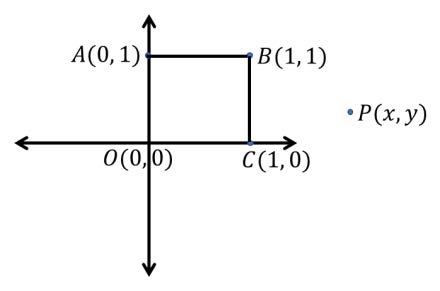(OP) 2 + (AP) 2 + (PC) 2 + (PB) 2=18

=> x2 + y2 + x2 + (y – 1) 2 + (x – 1) 2 + y2 + (x – 1) 2 + (y – 1) 2 =18

=> 4x2 + 4y2 – 4x – 4y=14

=> x2 + y2 – x – y=7/2

=> r=2

=> d = 4 => d2 = 16

Question 4: If loge (x+y) = 4xy. Find (d2y)/(dx2) at x = 0.

loge (x+y) = 4xy

or 1/((x + y)) [1 + (dy/dx)] = 4[x (dy/dx) + y]

or 1 + (dy/dx) = 4(x + y) [x (dy/dx) + y]⋯(i)

If x = 0, then y = 1

From (i)

1 + dy/dx = 4(0 + 1)[0 + 1] = 4

Or dy/dx = 3

From (i), again differentiate w.r.t. x

0 + (d2 y)/(dx2 ) = 4(x + y)[x (d2y)/(dx2 ) + 2 (dy/dx)] + 4[x (dy/dx) + y](1 + (dy/dx))

At x = 0, y = 1, dy/dx = 3

(d2 y)/(dx2) = 4(0 + 1)[0 + 2×3]+4[0 + 1](1 + 3) = 40

Therefore, (d2 y)/(dx2 ) = 40

Question 5: Find value of (5 – e2 ) for ellipse x2/8 + y2/4 = 1

x2/8 + y2/4 = 1

a2 = 8, b2 = 4

e = c/a = √((a2 – b2)/a2 ) = √(1-4/8) = √(1/2)

(5 – e2 ) = 5 – 1/2 = 9/2

Question 6: In a hospital, 89% has disease A and 98% has disease B, K% is the number of patients who has both, then K can’t be the subset of ____.

Answer: Least k =87, Max k = 89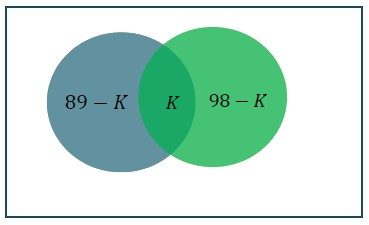Let total =100

n(A)=89, n(B)=98

max (n(A),n(B)) ≤ n(AUB) ≤ 100

98 ≤ n(A)+n(B)-n(A n B) ≤ 100

=> 98 ≤ 98 + 89- k ≤ 100

Least k =87

Max k = 89

Question 7: P(A) = p, P(B) = 2p, P(Exactly one out of A and B occurs) = 5/9. What will be the maximum value of p?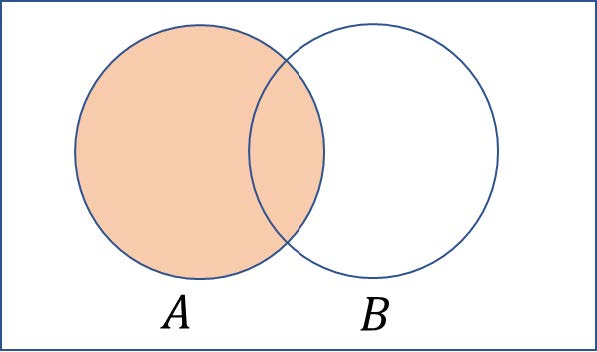Given: P(A) = p and P(B) = 2p

$$\begin{array}{l}P(\bar{A} \cap B) + P(\bar{B} \cap A) = \frac{5}{9}\end{array}$$

P(A)-P(A∩B)+P(B)-P(A∩B) = 5/9

3p-2P(A∩B) = 5/9

0 ≤ P(A∩B) ≤ p

For maximum value: 3p – 2p = 5/9

So, p = 5/9

Question 8: Evaluate

$$\begin{array}{l}\int_{\frac{-1}{\sqrt{2}}}^{\frac{1}{\sqrt{2}}}[(\frac{1-x}{1+x})^2 + (\frac{1+x}{1-x})^2 – 2]dx\end{array}$$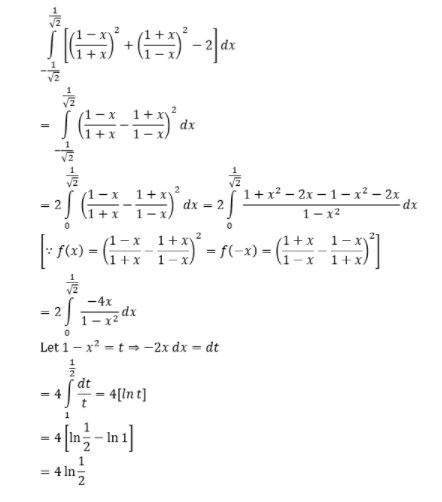Question 9: If cosx/(1+sinx) = |tanx|, then find the number of solutions in [0, 2π].

Case 1: tan x ≥ 0

cosx/(1+sinx ) = sinx/cosx

⇒cos2x = sin2x+sinx

⇒1-sin2x = sin2x + sinx

⇒2 sin2x + sin x – 1 = 0

⇒sin x = -1 ,1/2

sin x = 1/2 ⇒ x = π/6

sin x = -1 (not possible)

Case 2: tan x < 0

(Cos x)/(1+sinx) = -sin x/cos x

⇒cos2x = -sin2x – sinx

⇒1-sin2x = -sin2x – sinx

Sin x = -1 (Not possible)

Only one solution exists x = π/6

Question 10: Sum of infinite terms A, AR, AR2, AR3,…. is 15. If the sum of the square of these terms is 150, then find the sum of AR2, AR4, AR6, ⋯.

A + AR + AR2 + ⋯ = 15

⇒A/(1-R) = 15 ⋯(i)

Now, A2 + A2 R2 + A2 R4 + ⋯ = 150

⇒A2/(1-R2 ) = 150

⇒(A⋅A)/((1-R)(1 + R)) = 150

⇒15A/(1 + R) = 150

⇒A = 10(1 + R)⋯(ii)

Solving (i) & (ii):

10(1 + R)/(1-R) = 15

⇒R = 1/5

So, A = 12

AR2 + AR4 + AR6 + ⋯ = (AR^2)/(1-R2 ) = (12⋅1/25)/(1-1/25) = 1/2

Question 11: If

$$\begin{array}{l}arg(\frac{z-1}{z+1}) = \frac{\pi}{4}\end{array}$$
, then find equation of circle.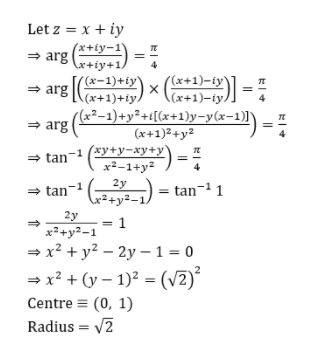Question 12: A wire of length 36 units is cut into two parts which are bent respectively to form a square of side x units and a circle of radius of r units. If the sum of areas of the square and the circle so formed is minimum, then find the circumference of the circle.

Answer: 2π x 18/(4 + π) = 36π/(4 + π)

Sum of perimeter of square and circle = 36

4x + 2πr = 36⇒2x + πr = 18

x = (18 – πr)/2 = 9 – πr/2 …..(i)

Sum of areas: S = x2 + πr2 ….(ii)

S = πr2 + (9 – πr/2) 2 , diff. w.r.to r

dS/dr = 2πr + 2(9 – πr/2)( – π/2)

dS/dr = 0

⇒r – 9/2 + πr/4

⇒r(π + 4)/4 = 9/2

⇒r = 18/(4 + π)

Circumference of circle = 2π x 18/(4 + π) = 36π/(4 + π)

Question 13: Solve

$$\begin{array}{l}\lim_{n \rightarrow \infty} \frac{1}{n}\sum_{r=0}^{2n-1}\frac{n^2}{n^2+4r^2}\end{array}$$

$$\begin{array}{l}\lim_{n \rightarrow \infty} \frac{1}{n}\sum_{r=0}^{2n-1}\frac{1}{1+\frac{4r^2}{n^2}}=\int_0^2 \frac{1}{1+(2x)^2}dx\end{array}$$

= [(1/2)] tan-14 – 0

Question 14: If

$$\begin{array}{l}\vec{a} . \vec{c} =3 , \vec{a} = \hat{i}+ \hat{j}+ \hat{k}, \vec{b} =\hat{j} – \hat{k}, \vec{a} \times \vec{c} =\vec{b}\end{array}$$
, Find
$$\begin{array}{l}[\vec{a} \ \; \vec{b} \;\; \vec{c}]\end{array}$$

$$\begin{array}{l}[\vec{a} \ \; \vec{b} \;\; \vec{c}]\end{array}$$
= -2

Given

$$\begin{array}{l}\vec{a} . \vec{c} =3 , \vec{a} = \hat{i}+ \hat{j}+ \hat{k}, \vec{b} =\hat{j} – \hat{k}, \vec{a} \times \vec{c} =\vec{b}\end{array}$$

This implies,

$$\begin{array}{l}(\vec{a} \times \vec{c})⋅.\vec{b} =\vec{b}.\vec{b}\end{array}$$

or

$$\begin{array}{l}[\vec{a} \ \; \vec{b} \;\; \vec{c}]\end{array}$$
= -2

Question 15: Evaluate

$$\begin{array}{l} \sum_{r=0}^{20} r^2(^{20}C_r)\end{array}$$

Answer: 20 ⋅ 21 ⋅ 218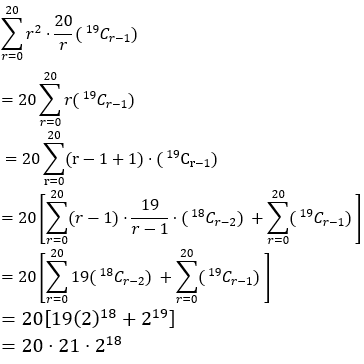Question 16: Solve (1 + y) tan2x + tan x (dy/dx) + y = 0

Answer: y tan x = -tan x + x + c

(1 + y) tan2x + tan x (dy/dx) + y = 0

tan x(dy/dx) + (tan2x + 1)y = -tan2x

dy/dx + (tan x + cot x )y = -tan x

I. F. =

$$\begin{array}{l}e^{\int{tan x + cotx)dx }}\end{array}$$

= e ln sec x + ln sin x

= e ln sec x . sin x

= tan x

• y tan x = -∫tan2 x dx
• y tan x = -∫(sec2 x-1) dx
• y tan x = -tan x + x + c

Question 17: If

$$\begin{array}{l}y = \frac{1}{1+x}+\frac{2}{1+x^2}+\frac{2^2}{1+x^4 }+….+\frac{2^100}{1+x^200}\end{array}$$
, then find y at x = 2.

$$\begin{array}{l}y = 1 + \frac{2^{101}}{1-2^{400}}\end{array}$$